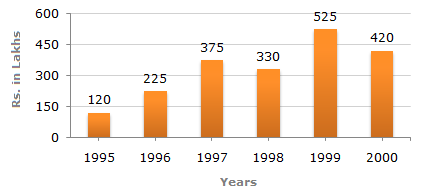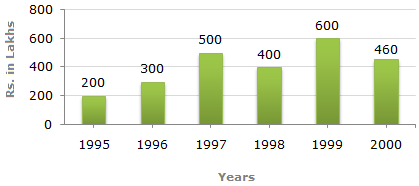# Data Interpretation - Bar Charts

Directions to Solve

Out of the two bar graphs provided below, one shows the amounts (in Lakh Rs.) invested by a Company in purchasing raw materials over the years and the other shows the values (in Lakh Rs.) of finished goods sold by the Company over the years.

Amount invested in Raw Materials (Rs. in Lakhs)Value of Sales of Finished Goods (Rs. in Lakhs)1.
The maximum difference between the amount invested in Raw materials and value of sales of finished goods was during the year?
1995
1996
1997
1998
Explanation:

The differences between the amount invested in raw material and the value of sales of finished goods for various years are:

For 1995 = Rs. (200 - 120) lakhs = Rs. 80 lakhs.

For 1996 = Rs. (300 - 225) lakhs = Rs. 75 lakhs.

For 1997 = Rs. (500 - 375) lakhs = Rs. 125 lakhs.

For 1998 = Rs. (400 - 330) lakhs = Rs. 70 lakhs.

For 1999 = Rs. (600 - 525) lakhs = Rs. 75 lakhs.

For 2000 = Rs. (460 - 420) lakhs = Rs. 40 lakhs.

Clearly, maximum difference was during 1997.

2.
The value of sales of finished goods in 1999 was approximately what percent of the sum of amount invested in Raw materials in the years 1997, 1998 and 1999?
33%
37%
45%
49%
Explanation:

Required percentage
 =600 x 100% (375 + 330 + 525)
= 48.78%49%.

3.
What was the difference between the average amount invested in Raw materials during the given period and the average value of sales of finished goods during this period?
Rs. 62.5 lakhs
Rs. 68.5 lakhs
Rs. 71.5 lakhs
Rs. 77.5 lakhs
Explanation:

Required difference

 = Rs.1 x (200 + 300 + 500 + 400 + 600 +460) 6

 - 1 x (120 + 225 + 375 + 330 + 525 + 420)lakhs 6

 = Rs.2460-1995lakhs 6 6

= Rs. (410 - 332.5) lakhs

= Rs. 77.5 lakhs.

4.
In which year, the percentage change (compared to the previous year) in the investment on Raw materials is same as that in the value of sales of finished goods?
1996
1997
1998
1999
Explanation:

The percentage change in the amount invested in raw-materials and in the value of sales of finished goods for different years are:

Percentage change in Amount invested in raw-material:

 For 1996 =(225 - 120) x 100% = 87.5%. 120

 For 1997 =(375 - 225) x 100% = 66.67%. 225

 For 1998 =(330 - 375) x 100% = -12%. 375

 For 1999 =(525 - 330) x 100% = 59.09%. 330

 For 2000 =(420 - 525) x 100% = -20%. 525

Percentage change in value of sales of finished goods:

 For 1996 =(300 - 200) x 100% = 50%. 200

 For 1997 =(500 - 300) x 100% = 66.7%. 300

 For 1998 =(400 - 500) x 100% = -20%. 500

 For 1999 =(600 - 400) x 100% = 50%. 400

 For 2000 =(460 - 600) x 100% = -23.33%. 600

Thus, the percentage difference is same during the year 1997.

5.
In which year, there has been a maximum percentage increase in the amount invested in Raw materials as compared to the previous year?
1996
1997
1998
1999
Explanation:

The percentage increase in the amount invested in raw-materials as compared to the previous year, for different years are:

 For 1996 =(225 - 120)% = 87.5%. 120

 For 1997 =(375 - 225)% = 66.67%. 225

For 1998 there is a decrease.

 For 1999 =(525 - 330)% = 59.09%. 330

For 2000 there is a decrease.There is maximum percentage increase in 1996.